## I- introduction:

• The problem of association rule extraction was introduced by Agrawal et al in 1993.
• Association rules are traditionally linked to the distribution sector because their main application is the "market basket analysis", which consists of searching for associations between products in sales receipts.
• This theme is nowadays considered as a part of unsupervised learning approaches, used in the field of data mining and knowledge extraction.
• An association rule is an implication relationship X → Y between two sets of articles X and Y.
• This rule indicates that transactions that contain articles in set X tend to contain articles in set Y.
• Rule: A rule defines the link between two itemsets X and Y that have no items in common. X→Y means that if X is in a transaction, Y can appear in the same transaction.
• Association rules can be applied to any sector of activity for which it is interesting to look for potential groups of products or services.
• Examples:
• if a customer buys a television then he will buy a VCR in a year.

## II- Basic concepts:

• Items: A limited list of atoms that describes a given field of application, they can be products, objects, patients, events, etc.

For example, for the application of the shopping basket, the list of items corresponds to the set of items available in the supermarket {Milk; Bread; Cheese; etc}

• Transaction: A set of items (minimum 1 item) labeled with a unique identifier, the items can belong to several transactions.

For example, two possible transactions that describe purchases in a supermarket are:  t1 = {Milk, Cheese, Bread} and t2 = {Milk, Cheese, Chocolate}

• The volume of the transaction: is the number of items contained in the transaction.
• Itemset: A succession of items expressed in a given and predefined order, the itemsets can belong to one or more transactions. An important notion for an itemset X is its support which refers to the number of observed transactions that contain it.

Example: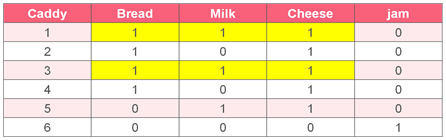The support of {Bread, Milk, Cheese} equal to 2.

• Rule Support: Probability of finding items or itemsets X and Y in a transaction. It is estimated by the number of times X and Y appear among all available transactions. It is between 0 and 1.
•  Sup (X→Y) = P(X ∩ Y)
• Rule Confidence: Probability of finding the item or itemset Y in a transaction, knowing that the item or itemset X is in the same transaction. It is estimated by the corresponding frequency observed (number of times that X and Y appear among all transactions divided by the number of times where X is found). It is between 0 and 1.
• Conf(X→Y) = P (Y / X) = P(X ∩ Y) / P(X) = Sup(X→Y) / Sup(X)

Example:

We have the association rule below:

R1: if {Bread, Milk} then {Cheese}In this rule, 100% is the confidence of the rule and 40% is the rule support.
"100% of transactions that contain Bread and Milk items also contain Cheese and 40% of transactions contain these three items »

"Good" rule = rule with high support and confidence

## III- APRIORI Algorithm:

Definition:

•  Apriori is an algorithm for frequent item set mining and association rule learning over transactional databases.
• It proceeds by identifying the frequent individual items in the database.

Inputs of the Apriori algorithm:

• A set of items I= {i1, i2, ......................, im}.
• A set of transactions (transaction base T) T= {t1, t2, ............, tn}.
• Each ti is a set of items t ⊂ I
• Each ti transaction has an identifier (TID).

Principle of the algorithm:

• It uses an iterative approach to find frequent itemsets in the transaction database and then generate association rules.
• It is based on the principle of Apriori: a subset of a frequent itemset must also be a frequent itemset.
• For example, if {I1, I2} is a frequent itemset then {I1} and {I2} must be frequent itemsets.
• More generally, subsets of k-1 items of a set of k frequent items are frequent.

Principle of the algorithm:

By using the property of frequency of the itemset, we can build the set of items:

• A set of k items can be built by joining a set of k-1 itemset with itself, and checking the frequency property.

Example:

We consider the itemset of 3 items below:

S = {(A,B,C), (A,B,D), (A,C,D), (A,C,E), (B,C,D)}

• S is joined with itself.
• (A,C,D,E) is not a set of 4 frequent items (because (C,D,E) is not in S).
• (A,B,C,D) is a set of 4 frequent items.

Functioning of the algorithm:

• The construction of frequent items is iterative: we first build frequent items containing only one item, then those containing 2 items, then those containing 3 items, ...
• We start by determining the frequent items of size 1, we note this set L1.
• Then, we build the set C2 of frequent candidate items of size 2: they are all the pairs built from the frequent items of size 1.
• The list of frequent items of size 2 (L2) is obtained by retaining only those elements of C2 whose support is greater than the threshold (the frequency property of the itemset).
• We then build C3, all the triplets of items whose 3 sub-pairs are in L2 and we only retain those whose support is higher than the threshold, which produces L3.
• We do the same thing until we find Li empty.

Example:

I = {bread, milk, cheese, jam, chocolate}

In this table, we give to each item a number in order                                                                                  to simplify the next stepsIteration 1:

We have the following transactions base. We suppose that Minimum support count = 2 Since we have the support of the itemset {4} = 1 which is lower than 2, so we will remove it in order to create L1.Iteration 2:

We will generate the list of itemsets of length 2, then remove the itemsets that have a support lower than the minimum support, to have a list of frequent itemsets of length 2.

Since we have the support of the itemset {1,2} = 1 which is lower than 2, so we will remove it in order to create L2.Iteration 3:

We will generate the list of itemsets of length 3, in order to create this time the list of frequent itemsets of length 3.We will apply the pruning method. First, we will start by dividing each set into sub-sets of length 2, then we will check if one of its sub-sets is not in the list of frequent candidate set items of length 2, in this case the set will be eliminated and we will keep only those sets that don’t contain a rare subset which are {1, 3, 5} and {2, 3, 5}, finally we will calculate their supports through the transaction database.Iteration 4:

We will generate the list of itemsets of length 4, in order to create the list of frequent itemsets of length 4.By pruning, this set must be eliminated since it has two rare sub-sets. And even if we compare its support with the minimum support, it will also be eliminated.Now, we don't have any more frequent itemsets so the algorithm is finished.

Frequent items:

The set L of the frequent subsets is the union of the sets L1,...LK.L1 = { 1,2,3,5 }                 L2 = { (1,3), (1,5), (2,3), (2,5), (3,5) }         L3 = { (1,3,5), (2,3,5) }

L = { 1,2,3,5,(1,3),(1,5),(2,3),(2,5), (3,5), (1,3,5), (2,3,5) }

Construction of rules:

For each frequent set, rules are constructed to verify the threshold constraint of confidence (Minconf).

We suppose that Minconf=100%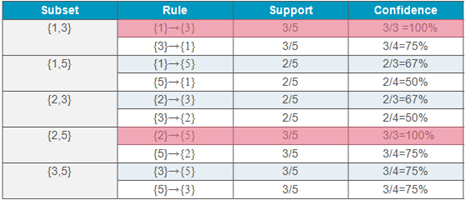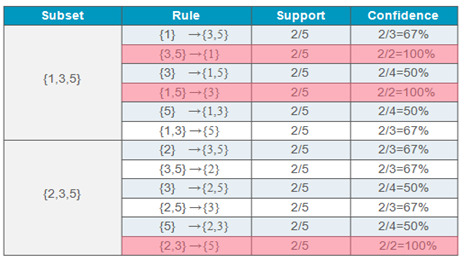These are the rules generated:In other words, the products that can be bought together are:

• 100% of transactions that contain the item bread also contain cheese
• 100% of transactions that contain the item milk also contain chocolate
• 100% of transactions that contain cheese and chocolate items contain also bread
• 100% of transactions that contain bread and chocolate items contain also cheese
• 100% of transactions that contain milk and cheese items contain also chocolate

The disadvantages of the Apriori algorithm:

• The calculation of supports is expensive.
• The generation of rules is expensive.
• The algorithm scans the database too many times, which reduces the overall performance. Due to this, the algorithm assumes that the database is permanent in the memory.

## IV- Frequent Pattern-Tree Growth Algorithm:

Definition:

• The Apriori algorithm performs several passes (scans) of the database, this can be very penalizing when we have voluminous data.
• In order to avoid this problem, Han et al. proposed a method to extract frequent itemsets without generating candidates, which is called FP-growth (Frequent Pattern growth), it was proposed in 1999.

Principle of the algorithm:

It consists first of compressing the database into a compact structure called FP-tree (Frequent Pattern tree), then dividing it into sub-projections of the database called conditional databases.

An FP-tree is a compact structure consisting of:

1. Tree: is composed of a null root, each node of the tree contains three pieces of information:
• The name of the item (item name): This is the item which represents the node.
• The number of the transaction’s occurrences where the portion of the path to this node is shown.
• A link to the next node in the tree (node-link). This is an inter-node link to other occurrences of the same item (having the same item-name) in other transaction sequences. This value is null if there is no such node.

1. Index: is a header table that contains the list of frequent items and points to the first occurrence of each element. Each input in this table contains:
• The name of the element (item name).
• The head pointer of the sequence of nodes with the same item-name.

The advantage of this representation of the data is that it is sufficient to follow the inter-node links to know all the frequent associations where the frequent element appears.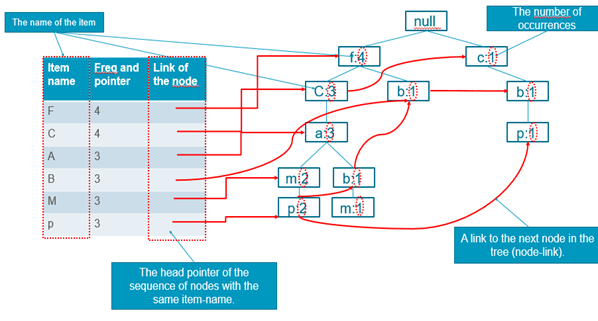Example:

• Construction of the FP tree:

Let's now consider the following transaction table:

We will count for each candidate the frequency, then we will put the items by ordered frequency and finally we will compare the support count for each candidate with min_supp.Now we will build an FP tree from this database.

For the transaction I2,I1,I5:

The sets of articles are considered in order of their value descending from the number of supports.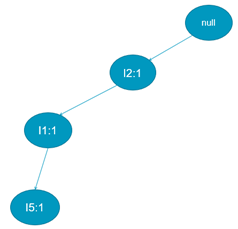For the transaction I2,I4:

We will add the articles of the transaction I2,I4 , if we find an article already added in the FP tree we will increment its support, else we will insert the new article and give it the value 1 of support.

In the FP tree below, we want to add the article I2, since it existed already we will increment its support from 1 to 2, then we will add the article I4.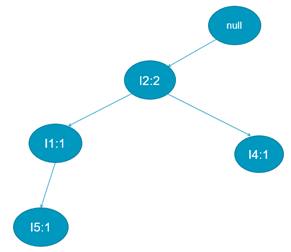For the transaction I2,I3:

Now we will add the transaction I2,I3, we will increment the support of I2 because it exists at the beginning of the FP tree, then we will insert the article I3, as shown in the FP tree below.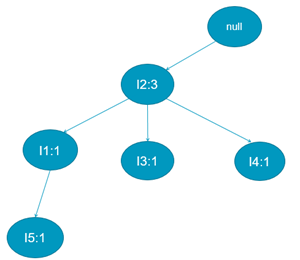For the transaction I2,I1,I4:

We will increment here the support of the article I2 and I1, also, then we will insert the article I4 in the FP tree because it doesn't exist after the article I1.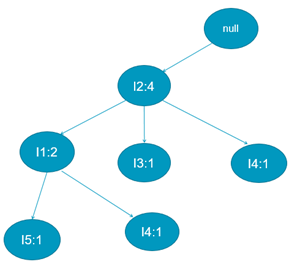For the transaction I1,I3:

As shown below, we will add the article I1 in the FP tree because it doesn’t exist at the beginning of the FP tree, then we will insert the I3 because it doesn't exist after I1.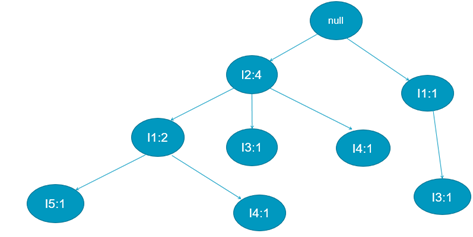For the transaction I2,I3:

Since we have at the beginning of the FP tree the article I2 we will just increment its support, and after I2 we can find the article I3 so we will also increment its support.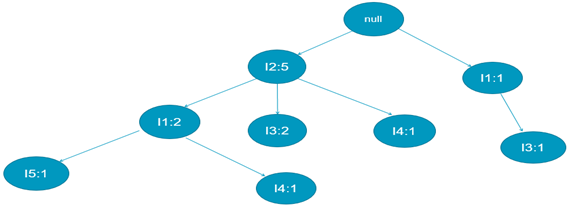For the transaction I1,I3:

Now we want to add the transaction I1, I3, for the article I1 it exists at the beginning of the FP tree, so we will increment its support to 2, then we have after I1 the article I3 that we need insert so we will increment its support also.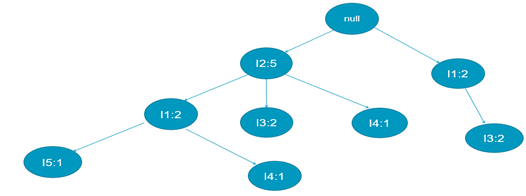For the transaction I2,I1,I3,I5:

We will apply the same thing to the transaction I2,I1,I3,I5, so we will increment the support of the article I2 from 5 to 6, then after I2 we can find I1 so we will also increment its support from 2 to 3, after that we will insert the article I3 because it doesn’t exist after I1, then we will insert after I3 the article I5.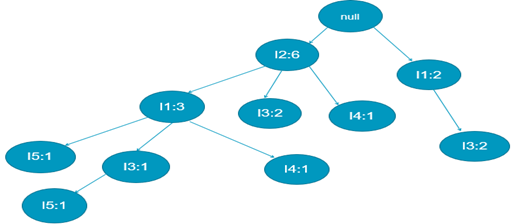For the transaction I2,I1,I3:

As shown in the FP tree below, we increment the support of the article I2 from 6 to 7 because we find it at the beginning of the FP tree, after I2 we can find the article I1 so we will increment its support from 3 to 4, and the same thing for the article I3 we will increment its support from 1 to 2.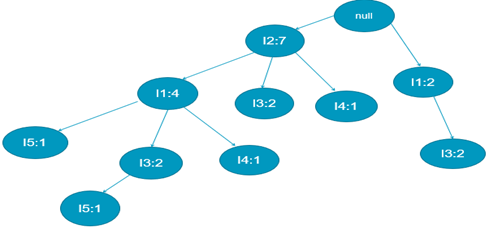Now we need to find the conditional basis and conditional FP Tree for each element.

To facilitate the tree crossing, an element header table is constructed so that each element points to its occurrences in the tree via a chain of node links.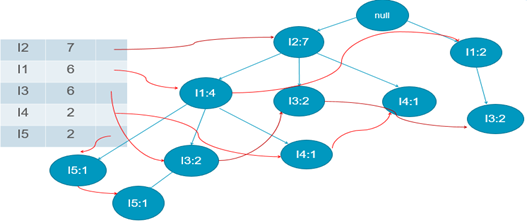• Conditional Pattern Base:
• Starting at the bottom of frequent-item header table in the FP-tree
• Traverse the FP-tree by following the link of each frequent item
• Accumulate all of transformed prefix paths of that item to form a conditional pattern base
• Conditional FP-tree: For each pattern base:
• Accumulate the count for each item in the base
• Construct the conditional FP-tree for the frequent items of the pattern base
• Frequent Patterns:
• For each frequent item, construct its conditional pattern-base, and then its conditional FP-tree
• Repeat the process on each newly created conditional FP-tree until the resulting FP-tree is empty, or it contains only one path—single path will generate all the combinations of its sub-paths, each of which is a frequent pattern.

For I5:

Conditional FP-tree: {I2: 2 I1: 2}

Conditional pattern base: {{I2,I1:1},{I2,I1,I3:1}}

Frequent patterns generated: {I2,I5:2}, {I1,I5:2}, {I2,I1,I5:2}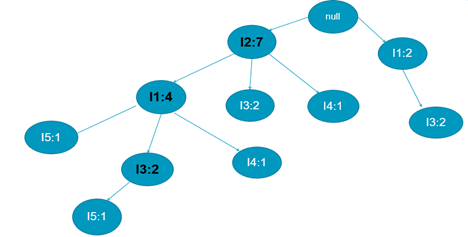For I4:

Conditional FP-tree: I2:2}

Conditional pattern base: {{I2,I1:1},{I2:1}}

Frequent patterns generated: {I2,I4:2}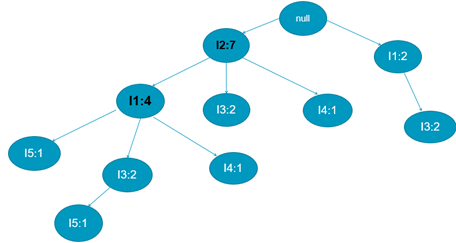For I3:

Conditional FP-tree: {I2:4,I1:2},{I1:2}

Conditional pattern base: {{I2,I1:2},{I2:2},{I1:2}}

Frequent patterns generated: {I2,I3:4}, {I1.I3:4}, {I2,I1,I3:2}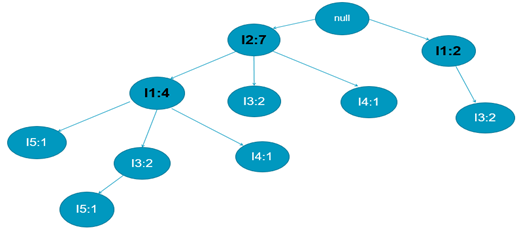For I1:

Conditional FP-tree: {I2:4}

Conditional pattern base: {{I2:4}}

Frequent patterns generated: {I2,I1:4}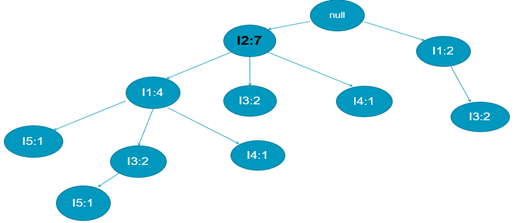The table below contains the conditional pattern base, the conditional FP-tree and the frequent patterns generated of each item.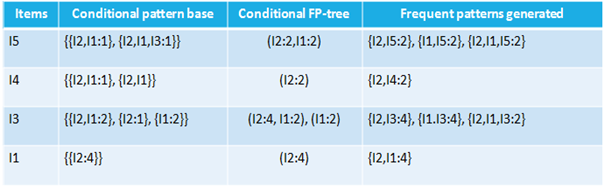• The major advantage of the algorithm is that it only scans the transaction database twice. The purpose of the first scan is to find the k-itemset and then sort the result to build the list of frequent items in T in order of frequency. The second scan is for the construction of the FP-tree structure.
• It’s much faster than Apriori.

• Even it has a compact structure, this does not guarantee, in the case if the transaction base is too large, that the entire FP-tree structure will hold in central memory.
• In addition, the construction of the FP-tree structure can be time consuming and could consume a lot of system resources.

## V- Evaluation of the rules :

Most association rules search algorithms use support and confidence measures using minimum thresholds to avoid uninteresting rules, but generating association rules can produce some of them uninteresting. So how to evaluate the association rules found?

There are several correlation measures, among them the lift test.

Lift:

Lift calculation

• The lift is a good measure of the performance of the association rule.
• The lift of a rule X-->Y is calculated as :

lift(X-->Y) = (sup(X U Y)/ N) / (sup(X)/ N*sup(Y)/ N ), where:

• N is the number of transactions in the transaction database.
• sup(X∪Y) is the number of transactions containing X and Y.
• sup(X) is the number of transactions containing X.
• sup(Y) is the number of transactions containing Y.

Lift Interpretation

• A lift greater than 1:
• Indicates a positive correlation.
• Among the baskets containing product X, we find more often the product Y only in all baskets.
• A lift equal to 1 indicates a zero correlation.
• A lift less than 1 indicates a negative correlation.

## VI- Conclusion :

• Association rule is an application of the form x->y that allows to express a correlation between the presence of an item and the presence of a set of items.
• There are many algorithms which help us to generate the association rules.
• Apriori is an algorithm that allows discovering the subsets of frequent items starting from those whose length is 1 and increasing the length.
• Apriori algorithm performs several passes (scans) of the database, which can be very penalizing when we have voluminous data.
• They proposed a method to extract frequent itemsets without generating candidates, which is called FP-growth (Frequent Pattern growth).
• In addition of the support and the confidence measures, there are several correlation measures, among them the lift test.

Author:

Kawtar Najmani

PhD Condidat At Faculty of Science Ben M'Sik